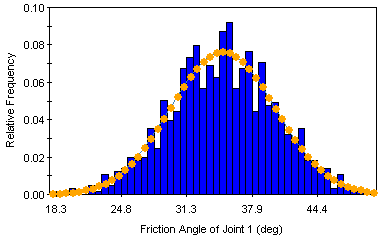Join one of our upcoming courses taking place around the world! Explore now

# Sampling

The Sampling options in the SWedge Project Settings dialog allow you to choose:

• Sampling Method
• Number of Samples
• Design Factor of Safety

for a Probabilistic Analysis in SWedge.

## Sampling Method

The Sampling Method determines how the statistical input distributions for the random variables you have defined for a Probabilistic Analysis, will be sampled. Two Sampling Methods are available in SWedge:

• Monte Carlo
• Latin Hypercube

NOTE: For both sampling methods, sequences of random numbers are utilized to generate the random samples. The generation of random numbers in SWedge is controlled by the Random Numbers option in the Project Settings dialog.

### MONTE CARLO METHOD

The Monte Carlo sampling technique uses random numbers to sample from the input data probability distributions. Monte Carlo techniques are commonly applied to a wide variety of problems involving random behaviour in geotechnical engineering.

Monte Carlo sampling of Normal distribution (1000 samples)### LATIN HYPERCUBE METHOD

The Latin Hypercube sampling technique gives comparable results to the Monte Carlo technique, but with fewer samples [Iman et.al. (1980), Startzman et.al. (1985)]. The method is based upon "stratified" sampling with random selection within each stratum. This results in a smoother sampling of the probability distributions. Typically, an analysis using 1000 samples obtained by the Latin Hypercube technique will produce comparable results to an analysis of 5000 samples using the Monte Carlo method [Hoek et.al. (1995)].

Latin Hypercube sampling of Normal distribution (1000 samples)## Number of Samples

The Number of Samples which will be generated for each random variable, for a Probabilistic Analysis. For example, if Number of Samples = 1000, then 1000 values of each input data random variable (e.g. Friction Angle of Joint 1) will be generated, according to the Sampling Method and statistical distribution for each random variable. The analysis will then be run 1000 times, and a safety factor calculated for each set of input data samples. This results in a distribution of safety factors, from which the Probability of Failure (PF) is calculated.

## Design Factor of Safety

The Design Factor of Safety is applicable for Probabilistic and Combination analyses and is used in the determination of Probability of Failure (i.e. PF = probability of Calculated FS < Design FS) and the number of failed wedges. The default value is 1.

The Design Factor of Safety does NOT influence the calculation of safety factor, it is only used as a failure threshold for post-processing of probabilistic or combination analysis results.